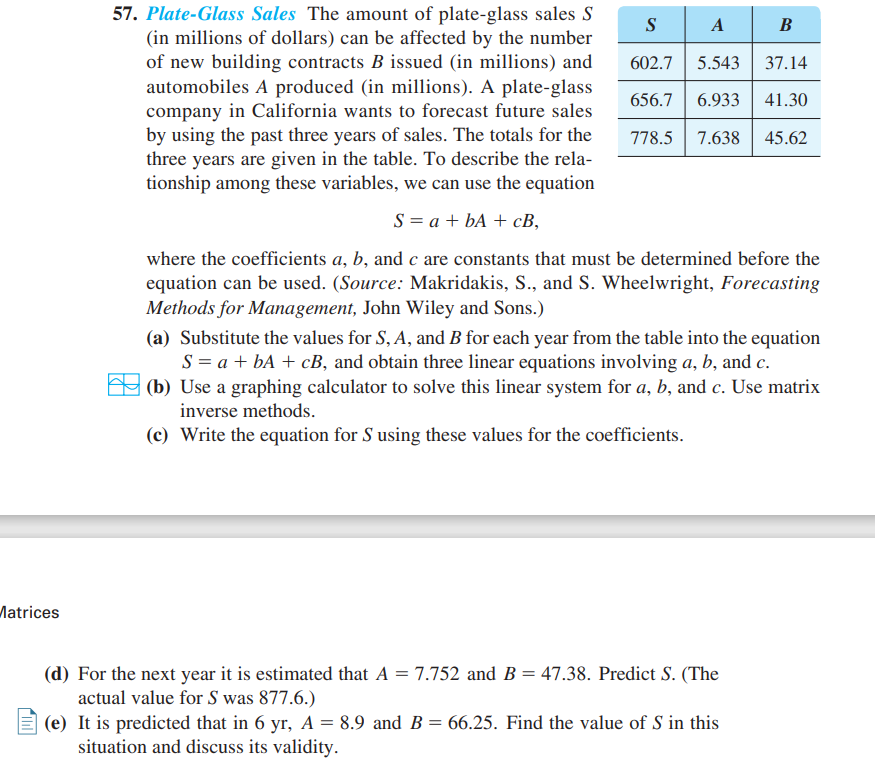### ¿Todavía tienes preguntas de matemáticas?

Pregunte a nuestros tutores expertos
Algebra
Pregunta57. Plate-Glass Sales The amount of plate-glass sales $$S$$ (in millions of dollars) can be affected by the number of new building contracts $$B$$ issued (in millions) and automobiles A produced (in millions). A plate-glass company in California wants to forecast future sales by using the past three years of sales. The totals for the three years are given in the table. To describe the rela- tionship among these variables, we can use the equation $$S = a + b A + c B$$where the coefficients $$a , b$$ , and $$c$$ are constants that must be determined before the equation can be used. (Source: Makridakis, S., and $$S$$ . Wheelwright, Forecasting Methods for Management, John Wiley and Sons.)

(a) Substitute the values for $$S , A$$ , and $$B$$ for each year from the table into the equation

$$S = a + b A + c B$$ , and obtain three linear equations involving $$a , b$$ , and $$c$$ .

(b) Use a graphing calculator to solve this linear system for $$a , b$$ , and $$c$$ . Use matrix inverse methods.

(c) Write the equation for $$S$$ using these values for the coefficients.

(d) For the next year it is estimated that $$A = 7.752$$ and $$B = 47.38$$ . Predict $$S$$ . (The actual value for $$S$$ was $$877.6$$ .)

(e) It is predicted that in $$6$$ yr, $$A = 8.9$$ and $$B = 66.25$$ . Find the value of $$S$$ in this situation and discuss its validity.

$$\left. \begin{array} { l } { \text { 57. (a) } 602.7 = a + 5.543 b + 37.14 c } \\ { 656.7 = a + 6.933 b + 41.30 c } \\ { 778.5 = a + 7.638 b + 45.62 c } \\ { \left. \begin{array} { l l } { \text { (b) } a \approx - 490.547 , b = - 89 , c = 42.71875 } \\ { \text { (c) } S = - 490.547 - 89 A + 42.71875 B } \\ { \text { (d) } S \approx 843.5 \quad \text { (e) } S \approx 1547.5 } \end{array} \right. } \end{array} \right.$$